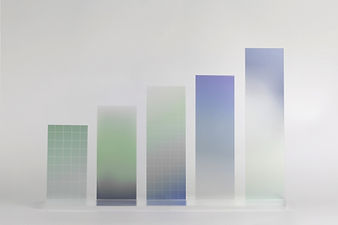top of page

# Data Visualization In Machine Learning

## What type of projects or assignments help looking for?​

• Assignment or Project Help

• Online Training and Mentorship

• New Idea or project

• Existing project that need more resources

## What is Data Visualization?

Data visualization is applied in practically every field of knowledge. Scientists in various disciplines use computer techniques to model complex events and visualize phenomena that cannot be observed directly, such as weather patterns, medical conditions or mathematical relationships.

## Types Of Data Visualization In Machien Learning?

• Line Plot

• Bar Chart

• Pie and Donut Charts

• Histogram Plot

• Scatter Plot

• Box Plot

• Heat Plot

### Line Plot

The simplest technique, a line plot is used to plot the relationship or dependence of one variable on another. To plot the relationship between the two variables, we can simply call the plot function:### Bar Chart

Bar charts are used for comparing the quantities of different categories or groups. Values of a category are represented with the help of bars and they can be configured with vertical or horizontal bars, with the length or height of each bar representing the value.### Pie Chart

A pie chart is a type of graph that represents the data in the circular graph. The slices of pie show the relative size of the data.### Histogram Plot

A histogram is a plot that lets you discover, and show, the underlying frequency distribution (shape) of a set of continuous data. This allows the inspection of the data for its underlying distribution (e.g., normal distribution), outliers, skewness, etc.### Scatter Plot

A scatter plot (also called a scatterplot, scatter graph, scatter chart, scattergram, or scatter diagram) is a type of plot or mathematical diagram using Cartesian coordinates to display values for typically two variables for a set of data.### Box Plot

A Box Plot is the visual representation of the statistical five number summary of a given data set.

A Five Number Summary includes:

• Minimum

• First Quartile

• Median (Second Quartile)

• Third Quartile

• Maximum### Heat Plot

A heat map (or heatmap) is a data visualization technique that shows magnitude of a phenomenon as color in two dimensions. The variation in color may be by hue or intensity, giving obvious visual cues to the reader about how the phenomenon is clustered or varies over spacebottom of page